﻿
Reach Us+44-7482-875032
Mathematically Describing Planetary Orbits in Two Dimensions

# Journal of Applied & Computational MathematicsOpen Access

All submissions of the EM system will be redirected to Online Manuscript Submission System. Authors are requested to submit articles directly to Online Manuscript Submission System of respective journal.

# Mathematically Describing Planetary Orbits in Two Dimensions

Ramanakumar V*
Chinmaya International Residential School, Nallur Vayal Post, Siruvani Road, Coimbatore, Tamil Nadu, India
*Corresponding Author: Ramanakumar V, Student, Chinmaya International Residential School, Nallur Vayal Post, Siruvani Road, Coimbatore, Tamil Nadu, India, Tel: +91 9962258783, Email: [email protected]

Received Date: Jul 08, 2017 / Accepted Date: Aug 20, 2018 / Published Date: Aug 21, 2018

Keywords: Natural satellites; Projectile; Motion; Planetary

#### Introduction

One of the biggest applications of mathematics is in the field of astrophysics, which is the study of the mechanical motion and behavior of celestial bodies . Using math, we can describe any celestial phenomena using numerical quantities which represent certain physical characteristics [2-5].

In this theoretical paper, mathematically describe the orbits of natural satellites and planets, and use this to calculate certain characteristics of the orbits of the planets within our solar system [6-9]. Once done this using mathematical method, calculated values to literature values already determined. The properties of a planetary orbit plan on investigating are:

1. The Cartesian form of an elliptical orbit

2. The Parametric form of an elliptical orbit

3. The Polar form of an elliptical orbit

4. The length of the orbit using various forms

5. The area covered by the ellipse of the planetary orbit

6. The volume carved out by a planet as it makes one full revolution around the sun.

At first glance, the motion of planetary bodies may seem complex and hard to understand . However, believe Math is a black and white subject that simplifies certain situations to numbers and equations. In this report, it attempt to simplify planetary revolutions through mathematical concepts.

Before proceeding with this investigation, it is important to introduce and layout the basic variables and quantities that will be used in this exploration . These quantities are included in the diagram below:

a: This represents the semi-major axis, which is the largest distance between the center of the ellipse and the curve of the orbit.

b: This represents the semi-minor axis, which is the shortest distance between the center of the ellipse and the curve of the orbit.

c: This is the distance between the center of the orbit and the focus of the orbit, which is where the Sun would be in a planetary orbit.

e: Although not included in the diagram, this quantity is known as eccentricity, which is the measure of the deviation of the shape of an orbit from that of a perfect circle (a measure of how elliptical an orbit is). The higher the eccentricity of an orbit, the more elliptical it is. It can be calculated using the simple formula: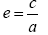(for an orbit into parametric 1).

After some research from wolframalpha.com and windows2universe.org, Tabulated these values for each planet. All values are given in astronomical units.

#### Cartesian Form of an Orbit

Given the fact that any planetary orbit is an ellipse, the Cartesian form will follow the basic form of an elliptical curve: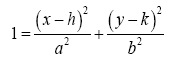Where h is the horizontal displacement of the curve, k is the vertical displacement of the curve a is the radius of the curve in the x direction, and b is the radius of the curve in the y direction.

Generally, in a planet’s orbital curve, there is no displacement in the y direction; the focus only shifts away from the origin in the x direction. Therefore, the value of k in the above equation for a planetary orbit becomes 0.

Now, we need to plug in the variables introduced in the previous section. Since c is the distance between the focus, which represents the origin O, and the center of the curve, this can be denoted as the horizontal displacement of the curve, so we can replace h with c. The variable a represents the semi-major axis, or the radius in the x direction, and will take the position of the variable a in the general form. The variable b represents the semi-minor axis, or the radius in the y direction, and will replace the variable b in the general form equation.

With the given replacements and adjustments, we get the general Cartesian form for the elliptical orbit of a planetary body: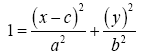#### Parametric Form of an Orbit

The parametric form of an equation expresses both variables in a function, x and y, in terms of another distinct variable. In this paper, we will refer to this variable as t (not to be confused with the variable for time).

We can convert our Cartesian equation for an orbit into parametric form in a few simple steps: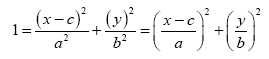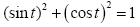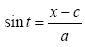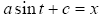and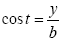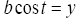#### Polar Form

Polar form equations are in terms of r, the distance from the origin, and θ, the angle between the line that connects a point on the curve and the origin and the positive x-axis.

It attempted to directly convert the Cartesian equation to the polar equivalent, but was quite unsuccessful. It decided to manipulate some key characteristics of an ellipse to derive the polar form. The two characteristics of an ellipse to keep in mind are as follows:

1. In an ellipse, with two foci (equidistant from the center/origin), the sum of the lines F1P and F2P are always equal to 2a, where P is any point on the ellipse (Figures 1 and 2).

Figure 1: Elliptical calculated using the simple formula: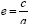2. If the first diagram is altered slightly, so that point P is on the semi-minor axis, both F1P and F2P are equal in length at a, and 2 right angle triangles are formed between the center/origin, both foci and point P. Using Pythagoras theorem, we can deduce that b2=a2-c2 (Figure 3).

Now, if we were to set F1 as the origin and let F2 some point on the positive x-axis denoted by (2c, 0), we get the in Figure 4.

We take w to be a vector connecting O and P. The vector connecting F2 and P is represented by w–2ci (the i is added to give direction to the magnitude 2c). The sum of the magnitude of both vectors is equal to 2a, according to the first statement made above: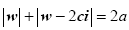Since the magnitude of w is equal to r (recall that r is the distance between the origin and the curve), we get: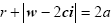Now we need to split the w–2ci vector into x and y components. We can denote the magnitude of the y component simply as y, but for the x component, we need to take into account 2ci, as this is in the x direction (Table 1). Therefore, the magnitude of the x component is x–2c:

Planetary Data
Planet Name a/AU b/AU c/AU e/AU
Mercury 0.3870 0.3788 0.0796 0.2056
Venus 0.7219 0.7219 0.0049 0.0068
Earth 1.0027 1.0025 0.0167 0.0167
Mars 1.5241 1.5173 0.1424 0.0934
Jupiter 5.2073 5.2010 0.2520 0.0484
Saturn 9.5590 9.5231 0.5181 0.0542
Uranus 19.1848 19.1645 0.9055 0.0472
Neptune 30.0806 30.0788 0.2587 0.0086

Table 1: Astronomical units.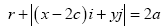The magnitude of the second vector is nothing but the square root of the sum of the squares of the x magnitude and y magnitude: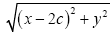.

Remember that in polar equations, x=γ cos θ and y=γ sin θ. Plugging these into the original equation gives: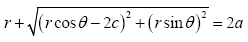We can rearrange this to get: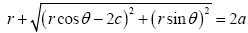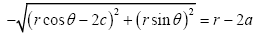(rcosθ-2c)2+(rsinθ)2=(r-2a)2

r2-4ar+4a2=r2(cosθ)2-4crcosθ+4c2+r2(sinθ)2

r2-4ar+4a2=r2((cosθ)2+(sinθ)2)-4cr cosθ+4c2

(cosθ)2+(sinθ)2=1

r2-4ar+4a2=r2-4cr cosθ+4c2

-4ar+4a2+4a2=-4cr cosθ+4c2

-ar+a2=-crcosθ+c2

-ar+cr cosθ=c2-a2

r(c cosθ-a)=c2-a2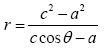From the second property discussed above (b2=a2-c2), we can further simplify to get the polar form of the curve: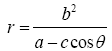We can plug in the values of a, b, and c from the above table of values for each planet and get the Cartesian, Parametric and Polar equations of their orbits (Table 2).

Planet Name Cartesian Equation Parametric Equation Polar Equation
Mercury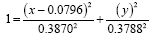0.3870 sin t+0.0796=x
0.3788 cost t=y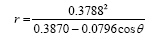Venus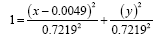0.7219 sin t+0.0049=x
0.7219 cost t=y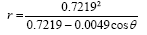Earth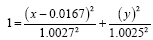1.0027 sin t+0.0167=x
1.0025 cost t=y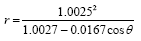Mars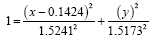1.5241 sin t+0.1424=x
1.5173 cost t=y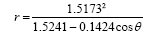Jupiter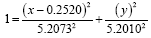5.2073 sin t+0.2520=x
5.2010 cost t=y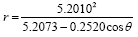Saturn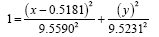9.5590 sin t+0.5181=x
9.5231 cos t=y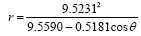Uranus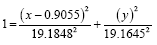19.1848 sin t+0.9055=x
19.1645 cos t=y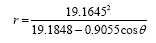Neptune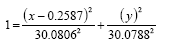30.0806 sin t +0.2587=x
30.0788 cos t=y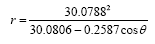Table 2: Values for each planet on Cartesian, Parametric and Polar equations of their orbits.

Using Desmos Online Graphing Calculator, it graphed the equations for the orbits of all 8 planets to get a two dimensional aerial view of the planetary orbits. The origin is the location of the Sun (Figures 5 and 6).:

Figure 5: (From outer to inner): Neptune, Uranus, Saturn, and Jupiter Zooming in gives a clearer picture of the inner planet orbits.

#### Length of the Curve of an Orbit

Now that we have the equations of the curve, we can calculate the length of this curve, which represents the length of each planet’s revolution. But first, let us derive the formula for length of a curve. If we have a graph of a function f(x), then the distance between any two distinct points on the curve is given by the following formula: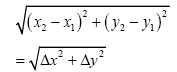If we were to bring these two points infinitely close to each other along the curve, the segment on the curve would be very close to a straight line, and Δx→0 and Δy→0. Due to this change, the above equation becomes: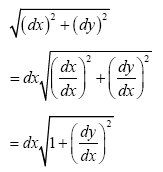If we set the limits [i, j] and integrate the function, we get the sum of the lengths of all the infinitesimally small lines which constitute the curve f(x):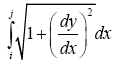This is the equation for the length of a curve in Cartesian form.

For parametric form, we go back to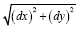and multiply and divide by dt to get: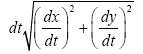Integrating this within some limit [k, l] will again give us the sum of the lengths of all the infinitesimally small lines which make up the curve f(x):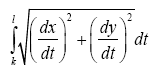For Polar form, it’s slightly trickier. We need to convert the polar function into a parametric function and then use the above equation for parametric equations. First, we need to separate x and y:

x=rcosθ

y=rsinθ.

Next we differentiate using the product rule: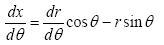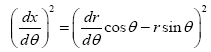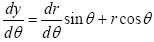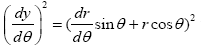.

Now that we have differentiated in parametric form, we can use the parametric formula for length of a curve by first simplifying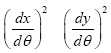: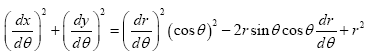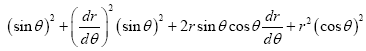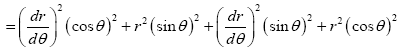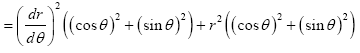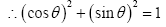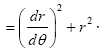Plugging this into the parametric equation formula, we get the general formula for the length of a polar curve: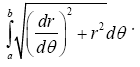With these three formulas, we can give the general equation for the length of a planetary elliptical orbit. Using Cartesian form: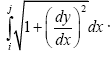To get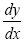, we implicitly differentiate our general Cartesian equation for a planetary orbit: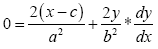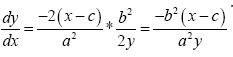Plugging this into the length of a curve formula gives us: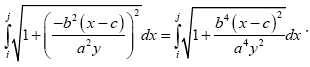In order to substitute for y2, we need to find y2 in terms of x: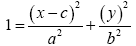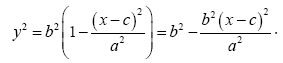Then, we need to simplify the denominator of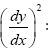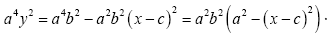Plugging this into the original equation, simplifying and setting the limits to [c-a,c+a] (to account for the horizontal displacement of the graph by c units), gives us: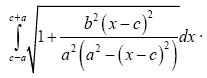Taking the limits [0, 2π] (since the period of both x and y are 2π, taking these limits will mean we get the length of the full curve since x and y go back to their original position), we can also find the length of the curve using the parametric form of the equation. First we need to find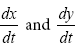x=asin t+c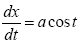y=bcos t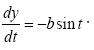Plugging this into the formula for length of a parametric curve, we get: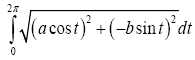Since we already have the general equation of r for a planetary orbit, we can also get the length of a planetary orbit if the equation is expressed in polar form: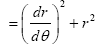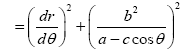In order to further simplify, you need to differentiate r with respect to θ using the quotient rule: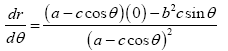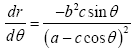Plugging this in, we get: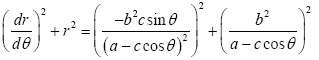We can input this simplification into our length of a polar curve formula. Integrating with the limits [0, 2π], we get: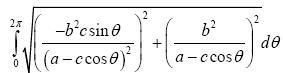We can also calculate the volume covered by a planet in a single orbit by multiplying the area of a circle with the same radius as the planet into the length formulas derived above, similar to how a prism’s volume is calculated by multiplying the cross sectional area and the length of the solid. Using this formula gives the volume as either: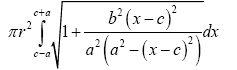For the Cartesian form equation or: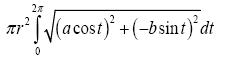For the Parametric form equation and: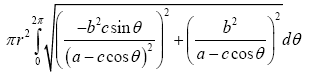For the Polar form equation, where r is the radius of the planet.

#### Mercury’s Orbit

To see whether my derivations were accurate, it plugged in already calculated values for the constants a, b, and c and compared the result with already calculated values found on reliable sources on the internet.

My first test was on the orbit of the planet Mercury, the first planet in the solar system which happens to have the largest value of e, eccentricity.

After in depth research I found that the approximated, widely accepted values for a, b are as follows:

a: 0.3870 astronomical units (a unit of distance used in astronomy which is equal to the average distance between the Earth and Sun) or 5.8 × 107 km

b: 0.3788 astronomical units or 5.7 × 107 km.

We can calculate the total distance covered by a planet in a single revolution based off of the length of a curve formula given above. We can choose to use either the Cartesian or the parametric form. Since the parametric form is slightly simpler to handle, let’s use that equation. Plugging in the above values gives us: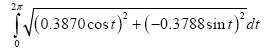Evaluating in a calculator gives a value of 2.4316 astronomical units. According to wolframalpha.com, the literature value of this distance is 2.4065, giving a reasonably low error percentage of 1.04%. I decided to tabulate my calculated values versus the literature values from the site for the rest of the planets (Table 3).

Planetary Orbit Lengths
Planet Name a/AU b/AU Calculated Value/AU Literature Value/AU Error Percentage
Mercury 0.3870 0.3788 2.4316 2.4065 1.04%
Venus 0.7219 0.7219 4.5358 4.5452 0.21%
Earth 1.0027 1.0025 6.3001 6.2826 0.28%
Mars 1.5241 1.5173 9.5762 9.5529 0.24%
Jupiter 5.2073 5.2010 32.7184 32.6714 0.14%
Saturn 9.5590 9.5231 60.0610 59.8779 0.31%
Uranus 19.1848 19.1645 120.5417 120.4874 0.05%
Neptune 30.0806 30.0788 189.0020 188.9070 0.05%

Table 3: Calculated values versus the literature values from the site for the rest of the planets.

My average error percentage is 0.29%. From this, I can conclude that my calculations and derivations are reasonably accurate.

#### Area Covered by the Elliptical Orbit of a Planet

If we visualize the elliptical orbit as a graph on a Cartesian plane, we can calculate the area covered by the curve, or in the words, the planet, in one complete revolution. To derive this expression, integrated the general equation of the curve and then added the limits [c-a,c+a]. First I made y the subject of the equation: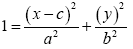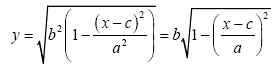Upon integration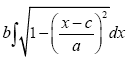We can use u- substitution to simplify the situation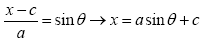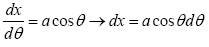Substituting gives us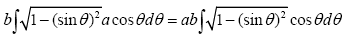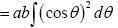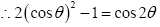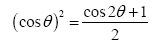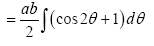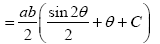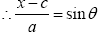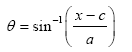Substituting for θ gives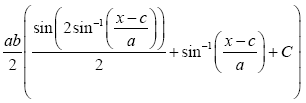After applying limits on the interval [c − a, c + a] , we can get the total area of the curve: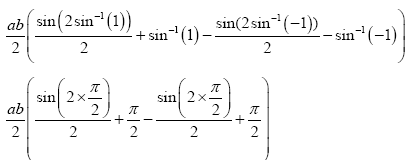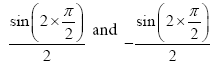both cancel out to give: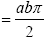Since this is the area under the hemisphere of the curve above the x-axis, we need to multiply by 2 to get the area of the full ellipse:

abπ

As expected, the above formula is the formula for the area of any ellipse, where a is the maximum radius and b is the minimum radius (Table 4).

Planet Name a/AU b/AU Area/AU^2
Mercury 0.3870 0.3788 0.4605
Venus 0.7219 0.7219 1.6372
Earth 1.0027 1.0025 3.1579
Mars 1.5241 1.5173 7.2650
Jupiter 5.2073 5.2010 85.0842
Saturn 9.5590 9.5231 285.9831
Uranus 19.1848 19.1645 1155.0593
Neptune 30.0806 30.0788 2842.4740

Table 4: Values for all the 8 planets.

#### Conclusion

This proof has become monumental for its ingenuity and its immense intellectual motion of Kepler concept. Therefore, the author considered it worthwhile to attempt to reach Mathcad conclusion via an alternate route, so that the beauty of the journey along this path be exposed to its full extent. Subsequently, based on the law of universal gravitation and his other great discovery, the concept of astrophysics, one-dimensional motion proved theoretically that planets do indeed prescribe elliptic trajectories around the sun. The position and velocity vectors at a particular instant of time discovered generating an ephemeris (right ascension and declination as a function of time) from the orbital elements in the real Two-dimensional situation.

#### References

Citation: Ramanakumar V (2018) Mathematically Describing Planetary Orbits in Two Dimensions. J Appl Computat Math 7: 414. DOI: 10.4172/2168-9679.1000414

Copyright: © 2018 Ramanakumar V. This is an open-access article distributed under the terms of the Creative Commons Attribution License, which permits unrestricted use, distribution, and reproduction in any medium, provided the original author and source are credited.

Select your language of interest to view the total content in your interested language

##### Recommended Journals
Viewmore
###### Article Usage
• Total views: 1580
• [From(publication date): 0-0 - Aug 26, 2019]
• Breakdown by view type
• HTML page views: 1519
• PDF downloads: 61

## Post your commentCan't read the image? click here to refresh
###### Peer Reviewed Journals

Make the best use of Scientific Research and information from our 700 + peer reviewed, Open Access Journals

International Conferences 2019-20

Meet Inspiring Speakers and Experts at our 3000+ Global Annual Meetings

Top